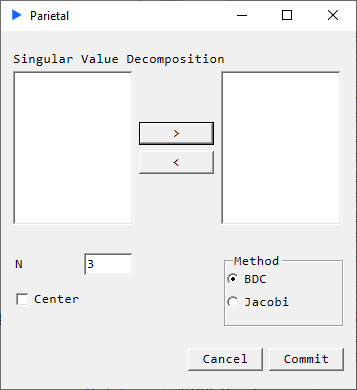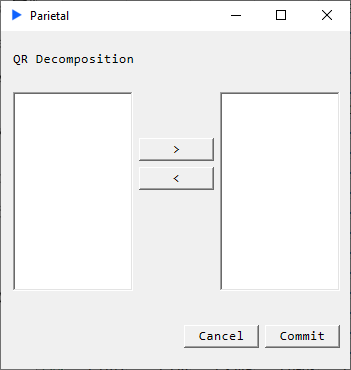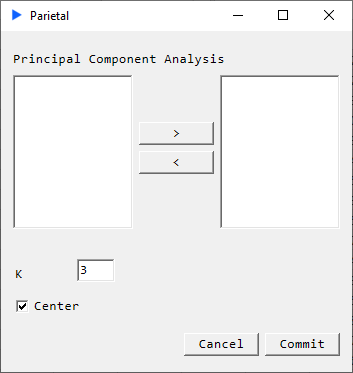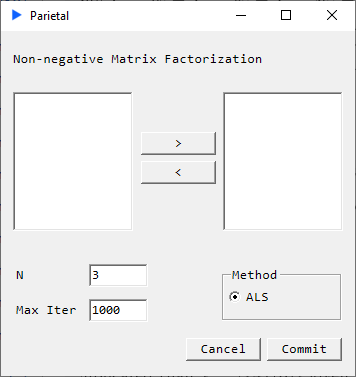# Matrix Ops

This section contains various matrix decomposition routines.

## SVD##### Description#

Singular Value Decomposition (SVD) performs a decomposition such that: $$M = U_{n} \Sigma_{n} V^{*}$$

#### Returns#

• Matrix $U_{n}$: Orthogonal matrix
• Matrix $\Sigma_{n}$: Ranked singular values
• Matrix $V^{*}$: Orthogonal matrix

## QR##### Description#

Performs a decomposition such that: $$M = QR$$ where $Q$ is an orthogonal matrix and $R$ is an upper triangular matrix.

#### Returns#

• Matrix Q: Orthogonal matrix
• Matrix R: Upper triangular matrix

## PCA##### Description#

Suppose we have a data matrix $X$. In Principal Component Analysis, we maximize the following relation:

$$F(W) = \frac{1}{n} \sum_{j=0}^{q-1} W_j^{\top} X^{\top} X W_j$$

subject to the constraint $W^{\top} W = I$ where $W$ is a matrix of $q$ orthonomal vectors.

#### Returns#

• Scores: Ranked projections
• Singular Values: Ranked eigenvalues
• W: Ranked columnwise coefficient matrix
• T2: Hotelling’s T-Squared
• % Variance: Percent variance explained by each component

## NNMF##### Description#

Suppose we have a matrix $V$ with no negative values and we want the following decomposition: $$V = W H$$ where $W$ and $H$ have only non-negative elements, this is known as Non-Negative Matrix Factorization.

#### Returns#

• $W_{m \mathrm{x} p}$
• $H_{p \mathrm{x} n}$